## 4. 1. 4 Linear Expressions and Functional Description

In the following sections the linear dependence of equations on different solution variables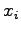is discussed. Typically, a solution variable is defined as a quantity on the underlying cell complex. Furthermore, each quantity value that is a solution value requires to be assigned a definite position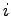in the solution vector.

In order to determine the position of the quantity associated with a given topological element, an index function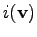is introduced. If more solution quantities are required, the function defermining the position of the solution within the vector can be obtained by different index functions (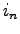and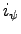for the quantities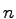and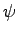).

In the following the residual expressions of discretized differential equations are formulated with linearized expressions. A residual expression is formulated and defines a dependence between single quantity values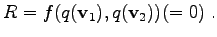(4.11)

Initially the quantity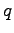may have any value and can not be neglected. The equation system may depend on the quantitywhich is evaluated on many different topological elements. For each of these elements an index functionis available that assigns each topological element a position in the solution vector. The formulation of the residual equation (4.11) can be written as follows: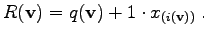(4.12)

This expression can be written using the lin() function as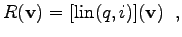(4.13)

where lin() is defined in the following way:(4.14)

In the following examples, the residual expressionsare replaced by their linearized analoga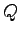, which implies that each quantity is added an increment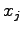, where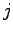is the position, given by the index function. This functionrepresents the position of the matrix column which is relevant for the quantityon the given element. If the residual equations are given in this manner, the solution consists of a vector of solution variableswhich are added to the quantitiesin order to obtain the final solution.

Michael 2008-01-16